Adapted for the Internet from:Why God Doesn't Exist
 A mathematician confusesa volume for an object

Predict architecture?

The mathematicians love to use their favorite word: predict. They do predictions all the time and call it 'science'. One of
their astrological claims is the power to 'predict' the architecture of a physical body by simply looking at an equation or,
in the alternative, to be able to synthesize architecture with a mathematical expression. Is this claim warranted?

Is an equation the same as an object?

It would seem elementary to teach that an equation or a volume is not the same as an object, but the mathematicians
have failed to realize this simple fact themselves:

“ the wavefunction is not simply a description of the object’s state, but that
it actually is entirely equivalent to the object” 

“ Solid space or Volume, which has three dimensions” 

If after 3000 years the mathematicians still don’t know the difference between an object and a concept, they have a

Intuitively, it is easy to see that an object cannot be inferred through an equation. Suppose you have a cube that is 2
meters per side (Fig. 1). If we multiply  2  meters  times  2   meters  times  2  meters, we  end  up  with  8  cubic meters
(written 8 meters ³). This we call the volume of the cube. A bigger cube, on the other hand may  have 3 meters per side,
giving us a volume of 27 cubic meters (3 meters * 3 meters * 3 meters = 27 meters ³). We can now replace the variable
component (the numbers 2 and 3) with an algebraic symbol, for example the letter X, and say that X³ = 8 meters³ when X
is equal to 2 meters, and that X³ = 27 meters³ when X is equal to 3 meters. Hence, we have represented the dimensions of
the geometrical figure we know as a cube in mathematical terms. According to mathematicians, the exponent 3 represents
the number of dimensions of the solid.

Now let’s try it in reverse. The mathematician writes an equation on the board in which you see the term X³. He clarifies that
X is equal to 2 meters (Fig. 2). What are we talking about: structure, location or motion? Do the 2 meters represent the length
of the side of a box, the distance between two objects, or the displacement of an object from here to there? In other words,
do the resulting 8 meters ³ represent the volume of a box, an imaginary itinerary or a perimeter in empty space?
 Fig. 2 Integration by aggregation              A. A still image of a cube.                          B. A still image of a rectan-     gular box
 Fig. 3    Integration by translationA.  A still image of a cube  B.  A movie of a moving cube
 Fig. 4    Al’s dimensional doughnuts
 Fig. 1   Mathematical representation of thevolume occupied by an object
 Fig. 2   Can you infer the shape or the number ofphysical dimensions from x³?

Objects we point to

The source of the problem is that the mathematicians routinely confuse the concept 'volume' for a physical object. For
example, a cube is an object and is confused for a concept when used, compared, or defined in some way. A volume,
on the other hand, will forever remain a concept. We use units such as ‘cubic meters’ (m³) to identify a volume. Now try
it with a cube. What meaning would it have to append units to a cube? If the prosecutors want the ET to know what a
cube is, they cannot present an expression such as 3 m³ and hope that the alien ‘understands’ what they are talking
about. The prosecutors have no choice but to point to a cube and say ‘cube.’ Only then can the ET visualize the object
in question and identify it with the sound. If the prosecutors want the ET to understand that the cube is 3D, they have
no choice but to introduce other objects such as a sphere, a square, or a chair and make a comparison. Conversely, a
volume makes no provision for shape. As far as Mathematics is concerned, we can have distinct geometrical figures
cubes, spheres and pyramids – with the same volume. A volume is all motion and no substance. The word volume
represents a dynamic concept. A volume is ‘extension’ in space: the amount of water that the rubber ducky displaces
inside the tub. From a conceptual point of view, the word volume means that the surface of a 3D object expanded to
'occupy' or 'displace' a certain amount of 'space'. (You have to think of space as an ocean here.)

“ The volume of a solid object is a numerical value given to describe the three-dimensional
concept of how much space it occupies... how much space an object displaces” 

And yet relativists get it wrong anyway...

" What is the shape of the universe? It is possible that it is Euclidean three-space: infinite
volume, with no straight path returning to meet itself. However, the universe might also
be finite in volume. You might be able to travel on a straight path and return to your
starting point. How is this possible?" 

[It’s possible when mathematical physicists don’t have the slightest clue of what they’re
talking about and confuse architecture with motion.]

We should, however, clarify that the word volume makes no provision for motion or for time. Swinging or translating a
plane does not constitute a volume. Neither is a volume a movie of an expanding solid. These techniques are merely
useful to calculate and conceptualize a volume. A volume is a finished product, a magnitude, and not the process of
integration itself. The magnitude of a volume is only valid for a cross-section of time (i.e., the last frame in a film). The
result of a calculation (e.g., 3 m³) may not be true a fraction of a second earlier or later. [Therefore, the doughnut I
illustrate below does not qualify as a volume.]

Let’s rephrase this lesson in scholarly, mathematical symbols so that the retards of relativity can also understand:

2D * 10 seconds  ≠  volume
3D * 10 seconds  ≠  hyper-volume
Capisci?

The shape of volume?

In regards to shape, does not tell us whether we are talking about a cube, a pyramid or a sphere.  For example can
you infer whether the 2 meters are oriented at 30º, 45º or 90º to each other from the quantity 8 meters³?   depicts a
volume – the amount of water the fish displaced. It tells you nothing about the fish.

Nevertheless, assuming you could deduce the dimensions or shape of the box you are trapped in from accurate
measurements of its sides and angles, it doesn’t follow that you can predict the dimensions or shape of the bag that
contains the box. If the Universe is 3-D as far as the telescope can see, how does it become a 4-D object called
space-time further down the road? How does a 3-D sphere acquire another physical dimension? Yet worse, how
does a star lose three dimensions and convert to a 0-D singularity?  Can these qualitative, architectural questions be

The Scientific Method requires relativists to define what they mean by object and by 4-D. This exercise does not involve
Math, but plain language. If space-time subsequently meets the criteria in these definitions, it qualifies as a 4-D object.
Math plays no role whatsoever in the entire process. There is no equation a mathematician can present in lieu of a
sphere to someone who has never seen a sphere. Mathematicians have no business defining physical objects in the
first place. Surely they cannot infer them from calculations because these are conceptual and qualitative issues that
have nothing to do with Mathematics. You don’t need to know Math to visualize an object. And you can't use Math to
arrive at a physical interpretation. That of a mathematician is certainly no truer than yours. This is easily demonstrated.
What does space-time look like? Can an equation tell you? Can any mathematician tell you? Nevertheless, the
mathematical physicists arrive at different physical interpretations for a given equation. If Math is so precise, the
equations should lead to the same qualitative results. To make matters worse for them, relativists have yet to
demonstrate that Math is the language of Physics. They don’t even have a definition of Physics they can agree on,
which again shows what little attention relativists pay to definitions. That’s where they should start.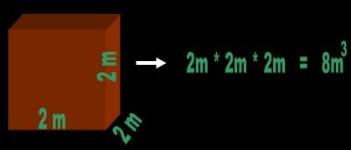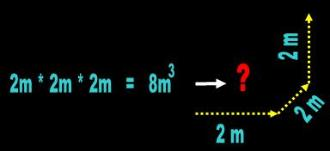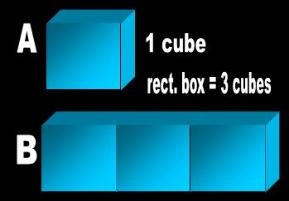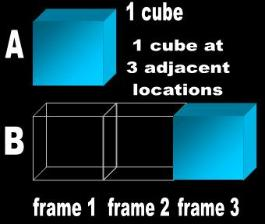Relativists allege that a 2D plane moving through the ‘dimension’ of time scans and thus ‘constructs’ a3D object. Thus it is that a 3D solid moving through or growing in time paints a 4D space-time. The following‘gedanken’ experiment refutes both these claims. Al’s doughnut is a film, not a still photograph!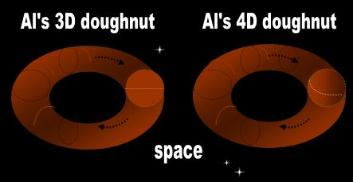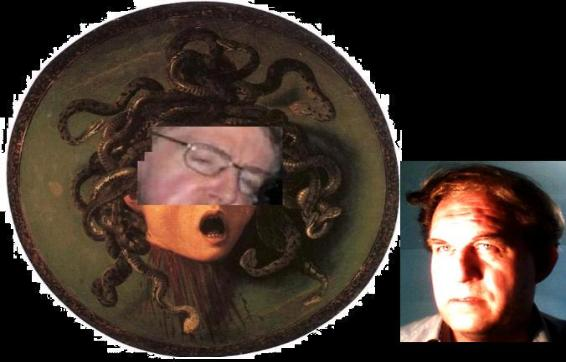I didn't dare look at myself in a mirror with my hair looking likethis, Bill. God! How awfully frightful! And I said to myself, like, whatwould my friend Al do in this case? So I eventually figured outthrough reasoning that I carry a sphere around my head bycalculating its volume.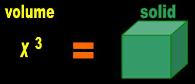?

________________________________________________________________________________________